# Analytics Memes & Humor

Just a list of comics and memes I have found related to Data Science

# Python: Confusion Matrix

## What is a confusion matrix?

A confusion matrix is a supervised machine learning evaluation tool that provides more insight into the overall effectiveness of a machine learning classifier. Unlike a simple accuracy metric, which is calculated by dividing the number of correctly predicted records by the total number of records, confusion matrices return 4 unique metrics for you to work with.

While I am not saying accuracy is always misleading, there are times, especially when working with examples of imbalanced data,  that accuracy can be all but useless.

Let’s consider credit card fraud. It is not uncommon that given a list of credit card transactions, that a fraud event might make up a little as 1 in 10,000 records. This is referred to a severely imbalanced data.  Now imaging a simple machine learning classifier running through that data and simply labeling everything as not fraudulent. When you checked the accuracy, it would come back as 99.99% accurate. Sounds great right? Except you missed the fraud event, the only reason to try to create the model in the first place.

A confusion matrix will show you more details, letting you know that you completely missed the fraud event. Instead of a single number result, a confusion matrix provides you will 4 metrics to evaluate. (note: the minority class – (in the case of fraud – the fraudulent events) – are labeled positive by confusion matrices. So a non-fraud event is a negative. This is not a judgement between the classes, only a naming convention)

TP = true positive – minority class (fraud) is correctly predicted as positive

FP = false positive – majority class (not fraud) is incorrectly predicted

FN = false negative – minority class (fraud) incorrectly predicted

TN = true negative – majority class (not fraud) correctly predicted

In matrix form: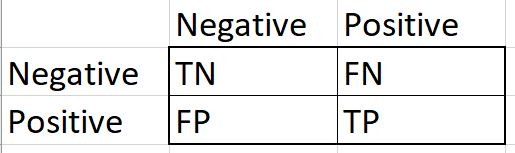To run a confusion matrix in Python, Sklearn provides a method called confusion_matrix(y_test, y_pred)

y_test = actual results from the test data set

y_pred = predictions made by model on test data set

so in a pseudocode example:

```model.fit(X,y)
y_pred = model.predict(X_test)```

If this is at all confusing, refer to my Python SVM lesson where I create the training and testing set and run a confusion matrix (Python: Support Vector Machine (SVM))

To run a confusion matrix in Python, first run a model, then run predictions (as shown above) and then follow the code below:

```from sklearn.metrics import confusion_matrix
confusion_matrix(y_test, y_pred)```

Output looks like this: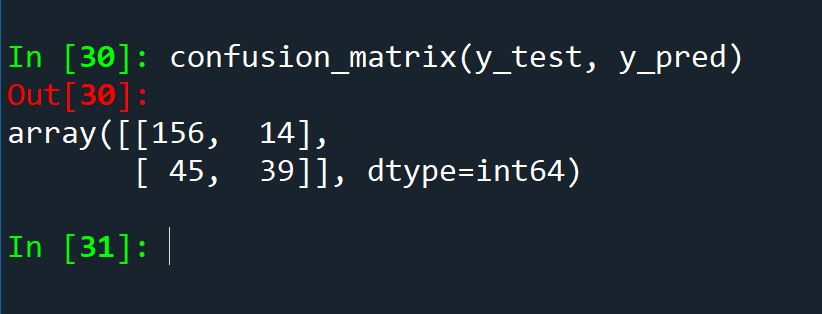Now, if you want to capture the TP, TN, FP, FN into individual variables to work with, you can add the ravel() function to your confusion matrix:

`TN,FP,FN,TP = confusion_matrix(y_test, y_pred).ravel()`

Thank you for taking the time to read this, and good luck on your analytics journey.

# Python: Support Vector Machine (SVM)

## Support Vector Machine (SVM):

A Support Vector Machine, or SVM, is a popular binary classifier machine learning algorithm. For those who may not know, a binary classifier is a predictive tool that returns one of two values as the result, (YES – NO), (TRUE – FALSE), (1 – 0).  Think of it as a simple decision maker:

Should this applicant be accepted to college? (Yes – No)

Is this credit card transaction fraudulent? (Yes – No)

An SVM predictive model is built by feeding a labeled data set to the algorithm, making this a supervised machine learning model. Remember, when the training data contains the answer you are looking for, you are using a supervised learning model. The goal, of course, of a supervised learning model is that once built, you can feed the model new data which you do not know the answer to, and the model will give you the answer.

## Brief explanation of an SVM:

An SVM is a discriminative classifier. It is actually an adaptation of a previously designed classifier called perceptron. (The perceptron algorithm also helped to inform the development of artificial neural networks).

The SVM works by finding the optimal hyperplane that can be used to discriminate between classes in the data set. (Classes refers to the label or “answer” column of each record.  The true/false, yes/no column in a binary set). When considering a two dimensional model, the hyperplane simply becomes a line that divides to the classes of data.

The hyperplane (or line in 2 dimensions) is informed by what are known as Support Vectors. A record from the data set is converted into a vector when fed through the algorithm (this is where a basic understanding of linear algebra comes in handy). Vectors (data records) closest to the decision boundary are called Support Vectors. It is on either side of this decision boundary that a vector is labeled by the classifier.

The focus on the support vectors and where they deem the decision boundary to be, is what informs the SVM as to where to place the optimal hyperplane. It is this focus on the support vectors as opposed to the data set as a whole, that gives SVM an advantage over a simple learner like a linear regression, when dealing with complex data sets.

## Coding Exercise:

Libraries needed:

sklearn

pandas

This is the main reason I recommend the Anaconda distribution of Python, because it comes prepackaged with the most popular data science libraries.

```from sklearn.model_selection import train_test_split
from sklearn.svm import SVC
from sklearn.metrics import metrics
from sklearn.metrics import confusion_matrix
import pandas as pd```

Next, let’s look at the data set. This is the Pima Indians Diabetes data set. It is a publicly available data set consisting of 768 records. Columns are as follows:

1. Number of times pregnant.
2. Plasma glucose concentration a 2 hours in an oral glucose tolerance test.
3. Diastolic blood pressure (mm Hg).
4. Triceps skinfold thickness (mm).
5. 2-Hour serum insulin (mu U/ml).
6. Body mass index (weight in kg/(height in m)^2).
7. Diabetes pedigree function.
8. Age (years).
9. Class variable (0 or 1).

pima_indians

now look at the data:Now keep in mind, class is our target. That is what we want to predict.

So let us start by separating the target class.

We use the pandas command .pop() to remove the Class column to the y variable, and the remained of the dataframe is now in the X

Let’s now split the data into training and test sets:

`X_train, X_test, y_train, y_test = train_test_split(X,y, test_size=0.33)`

Now we will train (fit) the model. This example I am using Sklearns SVC() model for an SVM example. There are a lot of SVMs available to try if you would like to explore deeper.

Code for fitting the model:

```model =SVC()
model.fit(X_train, y_train)```

Now using the testing subset we withheld, we will test our model

`y_pred = model.predict(X_test)`

Now to see how good the model is, we will perform an accuracy test.  This simply takes all the correct guess and divides them by total guesses.

As, you can seen below, we compare the y_pred (predicted values) against y_test (actual values) and we get .7677 or 77% accuracy. Which is not a bad model for simply using defaults.Let’s look at a confusion matrix to get just a little more in-depth infoFor those not familiar with a confusion matrix, this will help you to interpret results:

First number 151 = True Negatives — this would be the number of 0’s or not diabetics correctly predicted

Second number 15 = False Positives — the number of 0’s (non-diabetics) falsely predicted to be a 1

Third number 44 = False negatives — the number of 1’s (diabetics) falsely predicted to be a 0

Fourth number 44 = True Positives — the number of 1 (diabetics) correctly predicted.

So, the model correctly identified 44 out of the 59 diabetics in the test data, and misdiagnoses 44 out the 195 non diabetics in the data sample.

To see a video version of this lesson, click the link here: Python: Build an SVM# Ensemble Modeling

In the world of analytics,modeling is a general term used to refer to the use of data mining (machine learning) methods to develop predictions. If you want to know what ad a particular user is more likely to click on, or which customers are likely to leave you for a competitor, you develop a predictive model.

There are a lot of models to choose from: Regression, Decision Trees, K Nearest Neighbor, Neural Nets, etc. They all will provide you with a prediction, but some will do better than others depending on the data you are working with. While there are certain tricks and tweaks one can do to improve the accuracy of these models, it never hurts to remember the fact that there is wisdom to be found in the masses.

### The Jelly Bean Jar

I am sure everyone has come across some version of this in their life: you are at a fair or school fund raising event and someone has a large see-through jar full of jelly beans (or marbles or nickles). Next to the jar are some slips of paper with the instructions to “Guess the number of jelly beans in the jar you win!”

An interesting thing about this game, and you can try this out for yourself, is that given a reasonable number of participants, more often than not, the average guess of the group will perform better than the best individual guesser. Or in other words, imagine there are 200 jelly beans in the jar and the best guesser (the winner) guesses 215. More often than not, the average of all the guesses might be something like 210 or 190. The group cancels out their over and under guessing, resulting in a better answer than anyone individual.

### How Do We Get the Average in Models?

There are countless ways to do it, and researchers are constantly trying new approaches to get that extra 2% improvement over the last model. For ease of understanding though, I am going to focus on 2 very popular methods of ensemble modeling : Random Forests & Boosted Trees.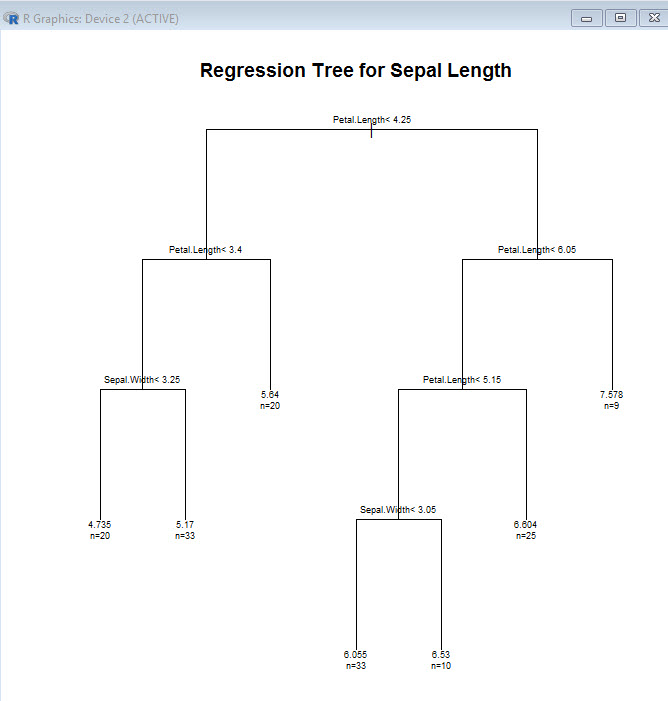Random Forests:

Imagine you have a data set containing 50,000 records. We will start by randomly selecting 1000 records and creating a decision tree from those records. We will then put the records back into the data set and draw another 1000 records, creating another decision tree. The process is repeated over and over again for a predefined number of iterations (each time the data used is returned to the pool where it could possibly be picked again).

After all the sample decision trees have been created (let’s say we created 500 for the sake of argument), the model then takes the mean or average of all the models if you are looking at a regression or the mode of all the models if you are dealing with a classification.

For those unfamiliar with the terminology, a regression model looks for a numeric value as the answer. It could be the selling price of a house, a person’s weight, the price of a stock, etc. While a classification looks for classifying answers: yes or no, large – medium – small, fast or slow, etc.

Boosted Trees:

Another popular method of ensemble modeling is known as boosted trees. In this method, a simple (poor learner) model tree is created – usually 3-5 splits maybe. Then another small tree (3-5 splits) is built by using incorrect predictions for the first tree. This is repeated multiple times (say 50 in this example), building layers of trees, each one getting a little bit better than the one before it. All the layers are combined to make the final predictive model.

### Oversimplified?

Now I know this may be an oversimplified explanation, and I will create some tutorials on actually building ensemble models, but sometimes I think just getting a feel for the concept is important.

So are ensemble models always the best? Not necessarily.

One thing you will learn when it comes to modeling is that no one method is the best. Each has their own strengths. The more complex the model, the longer it takes to run, so sometimes you will find speed outweighs desire for the added 2% accuracy bump. The secret is to be familiar with the different models, and to try them out in different scenarios. You will find that choosing the right model can be as much of an art as a science.

# Factor Analysis: Picking the Right Variables

Factor Analysis, what is it?

In layman’s terms, it means choosing which factors (variables) in a data set you should use for your model. Consider the following data set: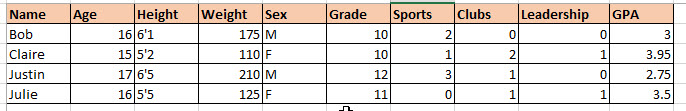In the above example, the columns (highlighted in light orange) would be our Factors. It can be very tempting, especially for new data science students, to want to include as many factors as possible. In fact, as you add more factors to a model, you will see many classic statistical markers for model goodness increase. This can give you a false sense of trust in the model.

The problem is, with too many poorly chosen factors, you model is almost guaranteed to under perform. To avoid this issue, try approaching a new model with the idea of minimizing factors, only using the factors that drive the greatest impact.

It may seem overwhelming at first. I mean where do you start? Looking at the list above, what do you get rid of? Well, for those who really love a little self torture, there are entire statistics textbooks dedicated to factor analysis. For the rest of us, consider some of the following concepts. While not an exhaustive list, these should get you started in the right direction.

## Collinearity

In terms of regression analysis, collinearity concerns itself with factors that have strong correlations with each other. In my example above, think Height and Weight. In general, as Height increases so does Weight. You would expect a 6’4 senior to easily outweigh a 4’11 freshman. So as one factor (Height) increases or decreases (Weight) follows in kind. Correlations can also be negative with one factor decreasing as another  factors increases or visa versa.The problem with these factors is that when used in a model, they tend to amplify their effect. So the model is skewed placing too much weight on what is essentially a single factor.

So what do you do about it?

Simply enough, in cases like this. You pick one. Height or Weight will do. In more complex models you can use mathematical techniques like Singular Value Decomposition (SVD), but I won’t cover that in this lesson.

I am also not going to cover any of the methods for detecting collinearity in this lesson, I will be covering those in further lessons. But it should be noted that a lot of times domain knowledge is really all you need to spot it. It doesn’t take a doctor to realize that taller people are generally heavier.

### But wait…

I know what you are thinking, what about the 250 lb 5’1 kid or the 120 lb 6’2 kid? Well if you have enough of these outliers in your data and you feel that being over or under weight is an important variable to consider, I would recommend using a proxy. In this case, you could substitute BMI (body mass index – a calculation based on height and weight) to replace both height and weight as factors.

## Stepwise

Stepwise regression is a method for determining which factors provide value to the model. The way it works (in the most basic definition I can offer) is you run your regression model with all your factors, removing the weakest factor each time (based on statistical evaluation methods like R^2 values and P values). This is done repeatedly until only high value factors are left in the model.

## Binning or Categorizing Data

Let’s say, looking at the data example above, our data covered all grades from 1-12. What if you want to look a kids in a two year period. You would want to bin the data into equal groups of 2: 1-2,3-4,5-6,7-8,9-10,11-12. You can now analyze the data in these blocks.

What if you wanted to measure the effectiveness of certain schools in the system. You might be wise to categorize the data. What that means is, we will take grades 1-6 and place them in one category (elementary), 7-8 in another(middle school), 9-12(high school).

## Incomplete Data

Imagine a factor called household income. This is a field that very likely may not be readily answered by parents. If there are only a few missing fields, some algorithms won’t be too affected, but if there are a lot, say 5%, you need to do something about it.

What are you options?

You could perform a simple mean or median replacement for all missing values, or try to calculate a best guess based on other factors. You could delete records missing this value. Or, as I often do, just toss this factor away. Most likely any value this adds to your model is going to be questionable at best. Don’t fall for the Big Data more is always better trap. Sometimes simplicity wins out in the end.

## Outliers and Erroneous Data

Outliers can really skew you model, but even worse, erroneous data can make you model absolutely worthless. Look out for outliers, question strange looking data. Unless you can come up with a real good reason why these should stay in your model, I say chuck the records containing them.

# R: Text Mining (Term Document Matrix)

There are a bounty of well known machine learning algorithms, both supervised (Decision Tree, K Nearest Neighbor, Logistical Regression) and unsupervised (clustering, anomaly detection). The only catch is that these algorithms are designed to work with numbers, not text. The act of using numeric based data mining methods on text is known as duo-mining.

So before you can utilize these algorithms, you first have to  transform text into a format suitable for use in these number based algorithms. One of the most popular methods people first learn is how to create a Term Document Matrix (TDM). The easiest way to understand this concept is, of course, to do an example.

```install.packages("tm") # if not already installed
library(tm)```

Now let’s create a simple vector of strings.

```wordVC <- c("I like dogs", "A cat chased the dog", "The dog ate a bone",
"Cats make fun pets")```

Now we are going to place the strings into a data type designed for text mining (from the tm package) called corpus. A corpus simply means the full collection of text you want to work with.

```corpus <- (VectorSource(wordVC))
corpus <- Corpus(corpus)
summary(corpus)```

Output:

``` Length Class Mode
1 2 PlainTextDocument list
2 2 PlainTextDocument list
3 2 PlainTextDocument list
4 2 PlainTextDocument list```

As you can see from the summary, the corpus classified each string in the vector as a PlaintextDocument.

Now let’s create our first Term Document Matrix

```TDM <- TermDocumentMatrix(corpus)
inspect(TDM)```

Output: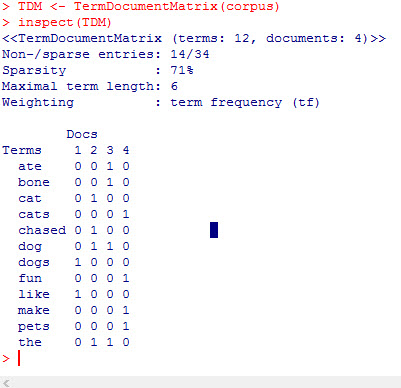As you can see, we now have a numeric representation of our text. Each row represents a word in our text and each column represents an individual sentence. So if you look at the word dog, you can see it appears once in sentence 2 and 3, (0110). While bone appears once in sentence 3 only (0010).

## Stemming

One immediate issue that jumps out at me is that R now sees the words cat & cats and dog & dogs as different words, when really cats and dogs are just the plural versions of cat and dog. Now there may be some more advanced applications of text mining that you would want to keep the two words separate, but in most basic text mining applications, you would want to only keep one version of the word.

Luckily for us, R makes that simple. Use the function tm_map with the argument stemDocument

`corpus2 <- tm_map(corpus, stemDocument)`

make a new TDM

```TDM2 <- TermDocumentMatrix(corpus2)
inspect(TDM2)```

Now you see only the singular of cat and dog exist in our list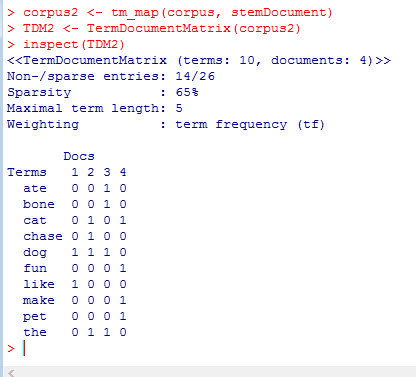If you would like, you can also work with the transpose of the TDM called the Document Term Matrix.

```dtm = t(TDM2)
inspect(dtm)```

dfsaI’ll get deeper into more pre-processing tasks, as well as ways to work with your TDM in future lessons. But for now, practice making TDMs see if you can think of ways that you can use TDMs and DTMs with some machine learning algorithms you might already know (decision trees, logistic regression).

# R: K-Means Clustering- Deciding how many clusters

In a previous lesson I showed you how to do a K-means cluster in R. You can visit that lesson here: R: K-Means Clustering.

Now in that lesson I choose 3 clusters. I did that because I was the one who made up the data, so I knew 3 clusters would work well. In the real world it doesn’t work that way. Choosing the right number of clusters is one of the trickier parts of performing a k-means cluster.

If you go over to Michael Grogan’s site, you will see he has a great method for figuring out how many clusters to choose. http://www.michaeljgrogan.com/k-means-clustering-example-stock-returns-dividends/

````wss <- (nrow(sample_stocks)-1)*sum(apply(sample_stocks,2,var))`
`for (i in 2:20) wss[i] <- sum(kmeans(sample_stocks,`
`centers=i)\$withinss)`
`plot(1:20, wss, type="b", xlab="Number of Clusters",`
`ylab="Within groups sum of squares")````

If you understand the code above, then great. That is a great solution for choosing the number of clusters. If, however, you are not 100% sure what is going on above, keep reading. I’ll walk you through it.

## K-Means Clustering

We need to start by getting a better understanding of what k-means clustering means. Consider this simplified explanation of clustering.

The way is works is each of the rows our data are placed into a vector.These vectors are then plotted out in space. Centroids (the yellow stars in the picture below) are chosen at random. The plotted vectors are then placed into clusters based on which centroid they are closest to.So how do you measure how good your clusters fit. (Do you need more clusters? Less clusters)? One popular metrics is the Within cluster sum of squares. R provides this as kmeans\$withinss. What this means is the distance the vectors in each cluster are from their respected centroid.

The goal is to get the is to get this number as small as possible. One approach to handling this is to run your kmeans clustering multiple times, raising the number of the clusters each time. Then you compare the withinss each time, stopping when the rate of improvement drops off. The goal is to find a low withinss while still keeping the number of clusters low.This is, in effect, what Michael Grogan has done above.

## Break down the code

Okay, now lets break down Mr. Grogan’s code and see what he is doing.

````wss <- (nrow(sample_stocks)-1)*sum(apply(sample_stocks,2,var))`
`for (i in 2:20) wss[i] <- sum(kmeans(sample_stocks,`
`centers=i)\$withinss)`
`plot(1:20, wss, type="b", xlab="Number of Clusters",`
`ylab="Within groups sum of squares")````

The first line of code is a little tricky. Let’s break it down.

`wss <- (nrow(sample_stocks)-1)*sum(apply(sample_stocks,2,var))`

sample_stocks – the data set

wss <-  – This simply assigns a value to a variable called wss

(nrow(sample_stocks)-1)  – the number of rows (nrow) in sample_stocks – 1. So if there are 100 rows in the data set, then this will return 99

sum(apply(sample_stocks,2,var)) – let’s break this down deeper and focus on the apply() function. apply() is kind of like a list comprehension in Python. Here is how the syntax works.

apply(data, (1=rows, 2=columns), function you are passing the data through)

So, let’s create a small array and play with this function. It makes more sense when you see it in action.

``` tt <- array(1:20, dim=c(10,2)) # create array with data 1 -20,
#10 rows, 2 columns
> tt
[,1] [,2]
[1,] 1 11
[2,] 2 12
[3,] 3 13
[4,] 4 14
[5,] 5 15
[6,] 6 16
[7,] 7 17
[8,] 8 18
[9,] 9 19
[10,] 10 20```

Now lets try running this through apply.

```> apply(tt, 2, mean)
 5.5 15.5```

Apply took the mean of each column. Had I used 1 as the second argument, it would have taken the mean of each row.

```> apply(tt, 1, mean)
 6 7 8 9 10 11 12 13 14 15```

Also, keep in mine, you can create your own functions to be used in apply

```apply(tt,2, function(x) x+5)
[,1] [,2]
[1,] 6 16
[2,] 7 17
[3,] 8 18
[4,] 9 19
[5,] 10 20
[6,] 11 21
[7,] 12 22
[8,] 13 23
[9,] 14 24
[10,] 15 25
```

So, what is Mr. Grogan’s doing with his apply function? apply(sample_stocks,2,var) – He is taking the variance of each column his data set.

``` apply(tt,2,var)
 9.166667 9.166667```

And by summing it: sum(apply(sample_stocks,2,var)) – he is simply adding the two values together.

``` sum(apply(tt,2,var))
 18.33333```

So, the entire first line of code using our data is:

wss <- (nrow(tt)-1)*sum(apply(tt,2,var))

wss <- (10-1) * (18.333)

```wss <- (nrow(tt)-1)*sum(apply(tt,2,var))
> wss
 165```

What this number is effectively is the within sum of squares for a data set that has only one cluster

### Next section of code

Next we will tackle the next two lines of code.

````for (i in 2:20) wss[i] <- sum(kmeans(sample_stocks,`
`centers=i)\$withinss)````

The first part is a for loop and should be simple enough. Note he doesn’t use {} to denote the inside of his loop. You can do this when your for loop is a single line, but I am going to use the {}’s anyway, as I think it makes the code a bit neater.

for (i in 2:20)  — a for loop iterating from 2 -20

for (i in 2:20) {

wss[i] <- }  – we are going to assign more values to the vector wss starting at 2 and working our way down to 20.

Remember, a single value variable in R is actually a single value vector.

```c <- 5
> c
 5
> c <- 7
> c
 5 7```

Okay, so now to the trickier code. sum(kmeans(sample_stocks, centers = i)\$withinss)

What he is doing is running a kmeans cluster for the data one time each for each value of centers (number of centroids we want) from 2 to 20 and reading the \$withinss from each run. Finally it sums all the withinss up (you will have 1 withinss for every cluster you create – number of centers)

### Plot the results

The last part of the code is plotting the results

````plot(1:20, wss, type="b", xlab="Number of Clusters",`
`ylab="Within groups sum of squares")````

plot (x, y, type= type of graph, xlab = label for x axis, ylab= label for y axis

## Let’s try it with our data

``` myData <- read.csv('cluster.csv')
StudentId TestA TestB
1 2355645.1 134 24
2 8718152.6 155 32
3 8303333.6 130 25
4 6352972.5 185 86
5 3381543.2 153 95
6 817332.4 153 81
> myData <- myData [,2:3] # get rid of StudentId column
TestA TestB
1 134 24
2 155 32
3 130 25
4 185 86
5 153 95
6 153 81```

Now lets feed this through Mr. Grogan’s code

````wss <- (nrow(myData)-1)*sum(apply(myData,2,var))`
```for (i in 2:20) {
wss[i] <- sum(kmeans(myData,```
```          centers=i)\$withinss)
```         }
`plot(1:20, wss, type="b", xlab="Number of Clusters",`
`ylab="Within groups sum of squares")````

Here is our output ( a scree plot for you math junkies out there)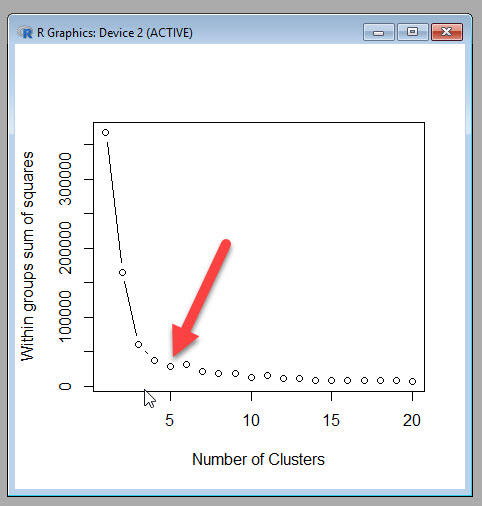Now Mr. Grogan’s plot a nice dramatic drop off, which is unfortunately not how most real world data I have seen works. I am going to chose 5 as my cut off point, because while the withinss does continue to decrease, it doesn’t seem to do so at a rate great enough to accept the added complexity of more clusters.

If you want to see how 5 clusters looks next to the three I had originally created, you can run the following code.

```myCluster <- kmeans(myData,5, nstart = 20)
myData\$cluster <- as.factor(myCluster\$cluster)
ggplot(myData, aes(TestA, TestB, color = cluster))
+ geom_point()```

5 Clusters3 Clusters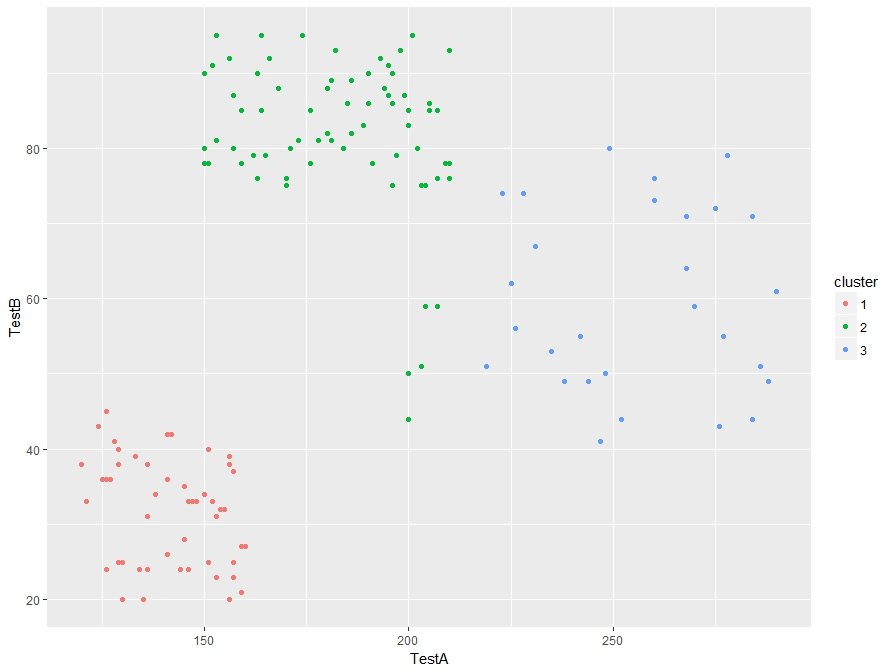I see some improvement in the 5 cluster model. So Michael Grogan’s trick for finding the number of clusters works.

# R: K-Means Clustering

Note: This is an introductory lesson with a made up data set. After you are finished with this tutorial, if you want to see a nice real world example, head on over to Michael Grogan’s website:

http://www.michaeljgrogan.com/k-means-clustering-example-stock-returns-dividends/

K Means Cluster will be our introduction to Unsupervised Machine Learning. What is Unsupervised Machine Learning exactly? Well, the simplest explanation I can offer is that unlike supervised where our data set contains a result, unsupervised does not.

Think of a simple regression where I have the square footage and selling prices (result) of 100 houses. Taking that data, I can easily create a prediction model that will predict the selling price of a house based off of square footage. – This is supervised machine learning

Now, take a data set containing 100 houses with the following data: square footage, house style, garage/no garage, but no selling price. We can’t create a prediction model since we have no knowledge of prices, but we can group the houses together based on commonalities. These groupings (clusters) can be used to gain knowledge of your data set.

I think seeing it in action will help.

Here is the data set: cluster

The data we will be looking at test results for 149 students.The task at hand is to group the students into 3 groups based on the test results. Now one thing any teacher will let you know is that some kids perform well in one subject and perhaps not so well in another. So we can’t simply group them on the score performance on one test. And when you are dealing with real world data, you might be looking at 20 -100 test/quiz scores per student.

So what we are going to do is let the computer decide how to group (or cluster) them.

To do so, we are going to be using K-means clustering. K-means clustering works by choosing random points (centroids). It then groups the data points around the centroids based which centroid the points are closest to.

## Let’s get started

```st <- read.csv(file.choose())

our dataNow let’s run the data through a Kmeans() algorithm

First, we are only going to want to focus on columns 2 and 3 in the data set since column 1 (studentID) is basically a label and provides no value in prediction.

To do this, we subset the data: st[,2:3] – which means I want all row ([,) and columns 2-3 (2:3])Now the code to make our clusters

```stCl <- kmeans(st[, 2:3], 3, nstart = 20)
stCl```

The syntax is kmeans(DATA, Number of clusters, Numbers of random starts)

Number of clusters I picked as 3 because I know this works well with the data, picking the right number usually takes a little trial and error in real life

Number of random starts is how many times you want the algorithm to be rerun (choosing new centroids each time) and choosing the result where the clusters are tightest.

Below is the output of our Kmeans – note the cluster means, this tells us the mean score for TestA and TestB set in each cluster.Hey, if you are a math junkie, this may be all you want. But if you are looking for some more practical value here, lets move on.

First, we need to add a column to our data set that shows our columns.

Now since we read our data from a csv, it is a data frame. If you can’t remember that, you can always run the command is.data.frame(st) to test it out.

Do you remember how to add a column to a data frame?

Well, there are multiple ways, but this is, in my opinion, the easiest way.

st\$cluster <- stCl\$cluster

```is.data.frame(st)
st\$cluster <- stCl\$cluster

Here is the resultNow with the clusters, you can group your students based their assigned cluster.

Technically we are done here. We have successfully grouped the students. But what if you want to make sure you did a good job. One quick check is to graph your work.

Before we can graph, we have to make sure our st\$cluster column is set as a factor, then using ggplot, we can graph it. (if you don’t have ggplot2 installed, you will need to run this line: install.packages(“ggplot2”)

```library(ggplot2)
st\$cluster <- as.factor(st\$cluster)
ggplot(st, aes(TestA, TestB, color = cluster)) + geom_point()```

And here is our output. The groups look pretty good.# R: Decision Trees (Regression)

Decision Trees are popular supervised machine learning algorithms. You will often find the abbreviation CART when reading up on decision trees. CART stands for Classification and Regression Trees.

In this example we are going to create a Regression Tree. Meaning we are going to attempt to build a model that can predict a numeric value.

We are going to start by taking a look at the data. In this example we are going to be using the Iris data set native to R. This data set

`iris`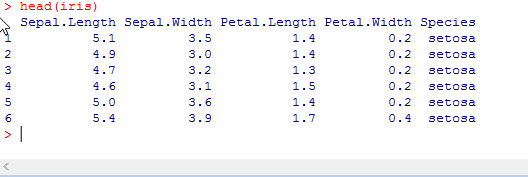As you can see, our data has 5 variables – Sepal.Length, Sepal.Width, Petal.Length, Petal.Width, and Species. The first 4 variables refer to measurements of flower parts and the species identifies which species of iris this flower represents.

In the Classification example, we tried to predict the Species of flower. In this example we are going to try to predict the Sepal.Length

In order to build our decision tree, first we need to install the correct package.

```install.packages("rpart")

library(rpart)```

Next we are going to create our tree. Since we want to predict Sepal.Length – that will be the first element in our fit equation.

```fit <- rpart(Sepal.Length ~ Sepal.Width + Petal.Length + Petal.Width+ Species,
method="anova", data=iris )```

Note the method in this model is anova. This means we are going to try to predict a number value. If we were doing a classifier model, the method would be class.

Now let’s plot out our model

```plot(fit, uniform=TRUE,
main="Regression Tree for Sepal Length")
text(fit, use.n=TRUE, cex = .6)```

Note the splits are marked – like the top split is Petal.Length < 4.25

Also, at the terminating point of each branch, you see and n= . The number following this is the number of elements from the data file that fit at the end of that branch.While this model actually works out pretty good, one thing to look for is over fitting. A good sign of that would be having a bunch of branches terminating with n values of 1 or 2. This means the model is tuned too much to the test data and when run up against a new set of data it will most likely result in poor predictions.

Of course we can look at some of the numbers if you are so inclined.Notice the xerror (cross validation error) gets better with each split. That is something you want to look out for. If that number starts to creep up as the splits increase, that is a sign you may want to prune some of the branches. I will show how to do that in another lesson.

To get a better picture of the change in xerror as the splits increase, let’s look at a new visualization

```par(mfrow=c(1,2))
rsq.rpart(fit)```

This produces 2 charts, 1rst on shows how R-Squared improves as splits increase (remember R-squared gets better as it approaches 1 so this model is improving with each spit)

The second chart shows how xerror decreases with each split. For models that need pruning, you would see the curve starting to go back up as the splits increase. Imagine is split 6 was higher than split 5.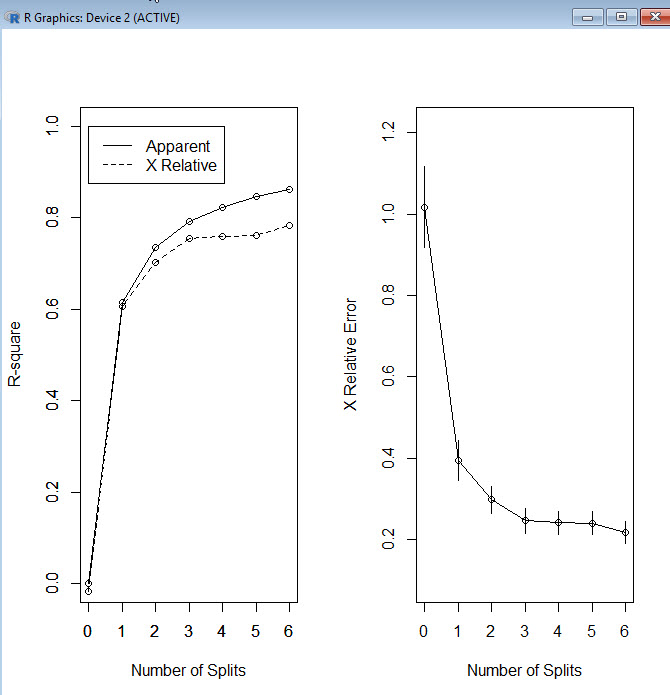Okay, so finally now that we know the model is good, let’s make a prediction.

```testData  <-data.frame (Species = 'setosa', Sepal.Width = 4, Petal.Length =1.2,
Petal.Width=0.3)
predict(fit, testData, method = "anova")```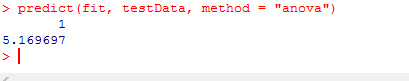So as you can see, based on our test data, the model predicts our Sepal.Length will be approx 5.17.